# Signal Processing

Signal processing consists of various manipulations or transformations performed on a measured signal. Origin provides a wide array of tools for your signal processing tasks.

Many tools provide a preview window with zoom control, and additional interactive features such as a vertical cursor for setting cutoff frequencies. Custom analysis settings for each tool can be saved as a theme for repeat use.The linear correlation result of Signal 1 and 2. The correlation tool in Origin provides both linear and circular correlation types, and an option to normalize the correlation coefficient.

## Smoothing and Filtering

### Smoothing

Origin offers multiple methods to smooth data:

• Savitzky-Golay
• FFT Filter
• Percentile Filter
• Lowess and Loess
• Binomial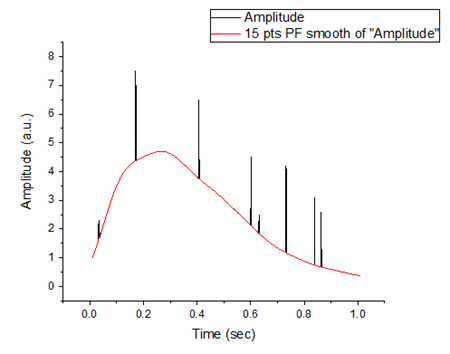The results generated from 50% Percentile Filter smoothing, useful for eliminating noise of abnormal amplitude. The Smooth tool in Origin provides several methods to remove noise, including Adjacent Averaging, Savitzky-Golay, Percentile Filter, FFT Filter, LOWESS, LOESS, and Binomial Method.

### Filtering

Filtering is commonly used in signal processing to filter out unwanted features and reveal components of interests.

FFT Filter

Origin supplies a FFT filter tool to select frequency components from an input signal by a specific filter type.

The following filter types are available:

• Low Pass
• Low Pass Parabolic
• High Pass
• Band Pass
• Band Block
• Threshold

Origin supplies an Infinite Impulse Response (IIR) filter to allow users design, analyze, and implement custom IIR digital filters.

A preview wizard is provided to enable real-time visualization of specified parameters and corresponding results.

The following filtering methods are available:

• Butterworth
• Chebyshev Type I
• Chebyshev Type II
• Elliptic

2D FFT Filter PRO

Origin supplies a 2D FFT filter to select desired frequency components from 2D signals in matrices.

The filters first perform a two-dimensional fast Fourier transform (2D FFT), then apply a frequency-domain filter window, and finally perform a 2D IFFT to convert them back to the spatial domain.

The following window types are available:

• Butterworth
• Ideal
• Gaussian
• Blackman

and there are four ways to specify a cutoff:

• Fraction
• Fourier Pixel
• Wavelength
• Frequency (Hertz)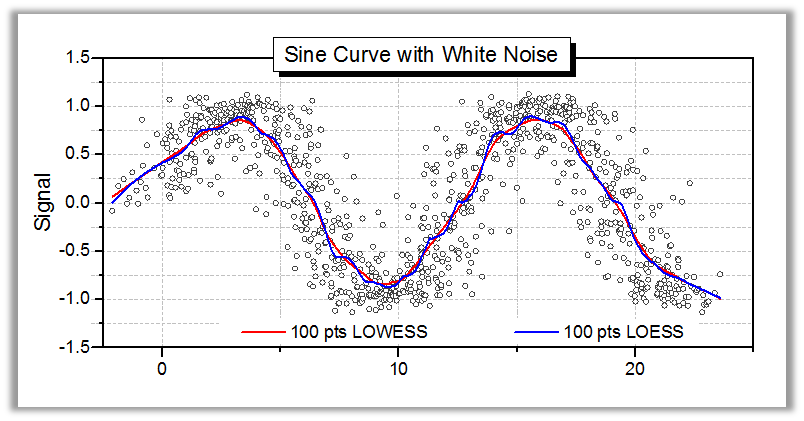The Smooth tool in Origin provides several methods to remove noise, including Adjacent Averaging, Savitzky-Golay, Percentile Filter, FFT Filter, LOWESS, LOESS, and Binomial Method. The graph shows results from LOWESS and LOESS smoothing, which are particularly useful for detecting trends in noisy data.The result of FFT filtering, with a Low-Pass filter type to block all frequency components above 100Hz. The FFT filter tool in Origin provides 5 types of filters: low-pass, high-pass, band-pass, band-block, and threshold. A preview panel provides real-time visualization of results and enables intuitive setting of cutoff frequencies or threshold.The IIR Filter tool in OriginPro can be used to design, analyze, and implement Infinite Impulse Response digital filters. Filter methods include Butterworth, Chebyshev Type I and II, and Elliptic filters. A preview panel is provided to enable real-time visualization of specified parameters and corresponding results.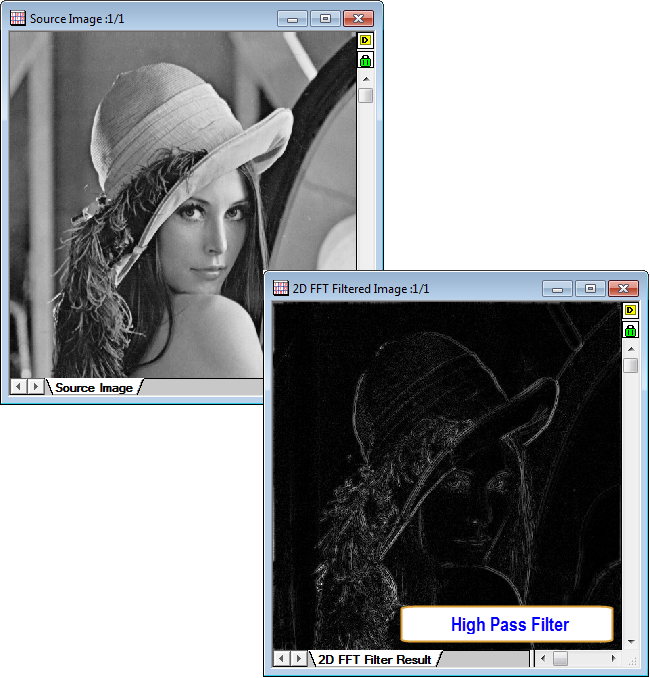The input matrix data and its 2D FFT filter result, with High-Pass filter type and Gaussian window. The 2D FFT filter tool in OriginPro provides 5 types of filters (low-pass, high-pass, band-pass, band-block, and threshold) and 4 types of filter window (Butterworth, Ideal, Gaussian, and Blackman). A preview panel provides the real-time visualization of the corresponding results.

## Signal TransformsWith the Fast Fourier Transform (FFT) tool, you can perform fast Fourier transforms on an input signal to obtain frequency data and complex transformed results. Additional computations include magnitude, amplitude, phase and power density. Five window types are supported: Rectangle, Welch, Triangular, Bartlett, Hanning, Hamming and Blackman. You can also transform the FFT results back to the original signal using the IFFT tool.The Short-Time Fourier Transform (STFT) tool in OriginPro performs time-frequency analysis of non-stationary signals. A Time-Frequency Analysis App using Continuous Wavelet Transform is also available.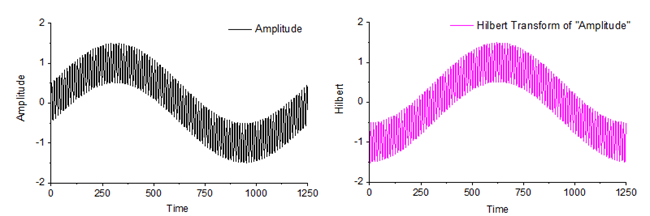Use the Hilbert Transform tool, to calculate the Hilbert transform results and /or the analytic signal which corresponds to the input signal.

### Fast Fourier Transform and Inverse Fast Fourier Transform (FFT/IFFT)

The Fast Fourier Transform tool in Origin is capable of computing the frequencies and complex transformed results with a wide selection of window options including:

• Triangular
• Rectangle
• Bartlett
• Welch
• Hanning
• Hamming
• Blackman

It also provides the magnitude, amplitude, phase, one/two sided power density, and other computation results. The power density estimation can be made by three different methods:

• MSA
• SSA
• TISA

### Short-Time Fourier Transform (STFT) PRO

The STFT tool in Origin is capable of performing STFT on real and complex signals and generate either complex STFT results or their amplitudes.

You can choose to specify the length of the moving window, steps that window advances, sampling interval and window type. The available window types are following:

• Rectangle
• Welch
• Triangular
• Bartlett
• Hanning
• Hamming
• Blackman
• Gaussian
• Kaiser

### Hilbert Transform PRO

The Hilbert Transform tool in OriginPro can calculate the Hilbert transformed results and the analytical representation of a real signal.

### 2D FFT/2D IFFT PRO

The 2D FFT tool in OriginPro performs forward 2D Discrete Fourier Transform (DFT) on matrix data to obtain the complex results and the amplitudes, phases, and powers derived from complex results.

You can choose to normalize the amplitude matrix and shift the DC component to the center of the result matrices.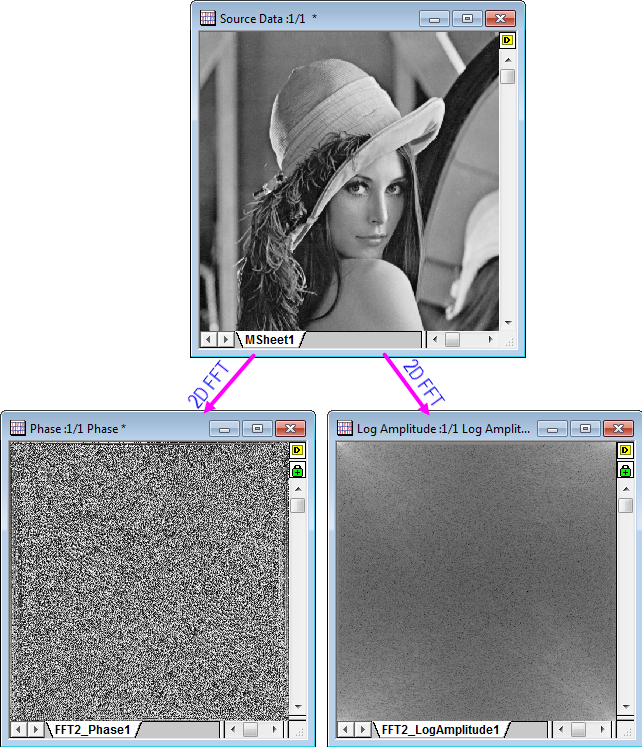With the 2D FFT Filters tool , you can apply a 2D FFT filter to signals in matrix or image forms. Five types of filters are available: Low Pass, High Pass, Band Pass, Band Block, and Threshold. There are four choices of window types: Butterworth, Ideal, Gaussian, and Blackman. Four cutoff methods are supported: Fraction, Fourier Pixel, Wave-length or Hertz.

## Wavelet Analysis PRO

### 1D Continuous Wavelet Transform PRO

The Continuous Wavelet Transform (CWT) tool in OriginPro is capable of computing wavelet coefficients for 1D real or complex signals. Three types of wavelet are supported:

• Morlet
• Derivative of Gaussian
• Mexican Hat

### 1D Discrete Wavelet Transform PRO

OriginPro also supports 2D wavelet decomposition and reconstruction. Both tools support standard wavelet families such as Haar, Daubechies and Biorthogonal wavelets of different orders.

1D Wavelet Decomposition PRO

The 1D Discrete Wavelet Transform (DWT) tool in OriginPro is capable of computing both approximation coefficients and detail coefficients according to the specified wavelet type and its order.With the Decomposition tool, you can decompose a 1D signal into approximation coefficents and detail coefficients. Two methods for end extension are available: periodic and zero-padded. Three types of wavelets are available, including Haar, Daubechies (N=2, 3, 4, 5, 6, 7, 8, 9, 10), and Biorthogonal (Nr.Nd=1.1, 1.3, 1.5, 2.2, 2.4, 2.6, 2.8, 3.1, 3.3, 3.5, 3.7).

1D Wavelet Reconstruction PRO

The 1D Inverse Discrete Wavelet Transform (IDWT) tool in Origin Pro is capable of reconstructing the 1D signal from the approximation and detail coefficients from a specified wavelet type.

Multi-Level 1D Wavelet Decomposition PRO

The 1D Multi-Level Discrete Wavelet Transform (MDWT) tool in OriginPro is capable of computing approximation coefficients and detail coefficients at different decomposition levels for specified wavelet type and its order.OriginPro provides several wavelet transform tools. This graph displays wavelet coefficients for a 1D signal computed using the Continuous Wavelet Transform (CWT) tool.

A Time-Frequency Analysis App is also available from our File Exchange site.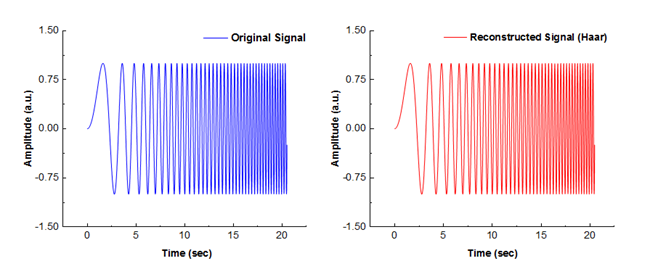With the Reconstruction tool, you can reconstruct a signal from its approximation coefficients and details coefficients. The coefficients are first upsampled and filtered using the wavelet type applied during signal decomposition. Three types of wavelets are available, including Haar, Daubechies (N=2, 3, 4, 5, 6, 7, 8, 9, 10), and Biorthogonal (Nr.Nd=1.1, 1.3, 1.5, 2.2, 2.4, 2.6, 2.8, 3.1, 3.3, 3.5, 3.7).With the Multi-Scale Wavelet Decomposition tool, you can decompose the input signal into approximation coefficients and details coefficients at different levels. Three types of wavelets are supported: Haar, Daubechies (N=2, 3, 4, 5, 7, 8, 9), and Biorthogonal (Nr.Nd=1.1, 1.3, 1.5, 2.2, 2.4,2.6, 3.1, 3.3, 3.5, 3.7).In Origin, you can use the 2D Wavelet Decomposition tool to decompose a 2D signal into its approximation coefficients, and horizontal/vertical/diagonal details coefficients. On the other side, you can reconstruct a 2D signal from those coefficients using 2D Wavelet Reconstruction tool. Three types of wavelets are supported: Haar, Daubechies (N=2, 3, 4, 5, 7, 8, 9), and Biorthogonal (Nr.Nd=1.1, 1.3, 1.5, 2.2, 2.4,2.6, 3.1, 3.3, 3.5, 3.7).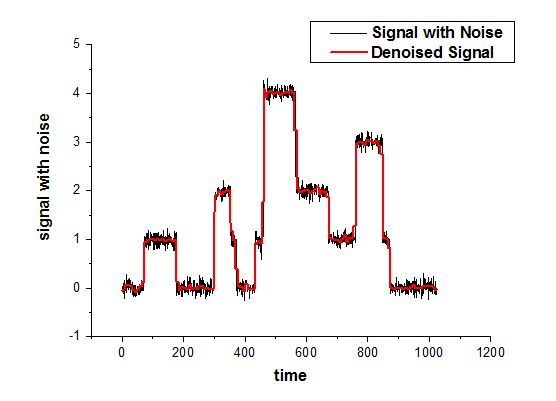With the Wavelet Denoising tool, you can remove noise from signals by applying thresholding to detail coefficents in wavelet decompositon and reconstruciton processes. You can choose the wavelet type, extension mode and method for thresholding.With the Wavelet Smoothing tool, you can remove noise from signals by cutting off the detail coefficients of the signal. You can choose a wavelet type to be used for decomposition and reconstruction and specify the end extension mode.

### 2D Wavelet Transform PRO

2D Wavelet Decomposition PRO

The 2D Discrete Wavelet Transform (DWT2) tool is capable of decomposing a 2D signal that is saved in a matrix into its approximation coefficients, horizontal detail coefficients, vertical detail coefficients and diagonal detail coefficients according to a specified wavelet type.

2D Wavelet Reconstruction PRO

The 2D Inverse Discrete Wavelet Transform (IDWT2) tool can reconstruct the 2D signal from approximation coefficients, horizontal, vertical and diagonal detail coefficients from a specified wavelet type.

### Wavelet Denoising and Smoothing PRO

OriginPro supplies Wavelet Denoising (WTDENOISE) tool to remove noise from signals as well as Wavelet Smoothing (WTSMOOTH) tool to smooth signals using wavelet transform.

Wavelet Denoising PRO

The Wavelet Denoising tool removes signal noise based on multi-level 1D discrete wavelet transform. The degrees of denoising can be controlled by adjusting the level for the wavelet decomposition, the wavelet type and the method to perform the thresholding.

Wavelet Smoothing PRO

The Wavelet Smoothing (WTSMOOTH) tool smoothes signals based on multi-level 1D discrete wavelet transform. The degrees of smoothing is controlled by the cut-off percentage of detail coefficients.

### Convolution

The convolution of two data sets is a general process that can be used for various types of data smoothing, signal processing, or edge detection. Its main purpose is to include the effect of system response on a signal.

### Correlation

Origin supports 1D and 2D correlation to detect the correlation between a pair of signals.

 Correlation 2D Correlation PRO

### Coherence PRO

Coherence- the degree of linear dependency of two signals- is evaluated by testing whether the signals contain similar frequency components.

### Envelope Curves PRO

An envelope curve traces the crests and troughs of a periodic signal.

• Choose upper, lower or both
• Smoothing option during envelope detectionWith the Envelope tool, you can obtain the upper, lower , or both envelopes of the source data with additional control over the smoothness of envelope(s).

### Decimation PRO

Decimation is used to reduce the number of elements in an input sequence. Every N samples are merged into one. Two filters are available:

• Moving Average
• Finite Impulse Response (FIR)

The Rise Time gadget can be used to analyze the rising and falling stages of a step-like signal.

Three methods of finding the rise/fall time are supported:

• Linear search
• Histogram
• Largest triangle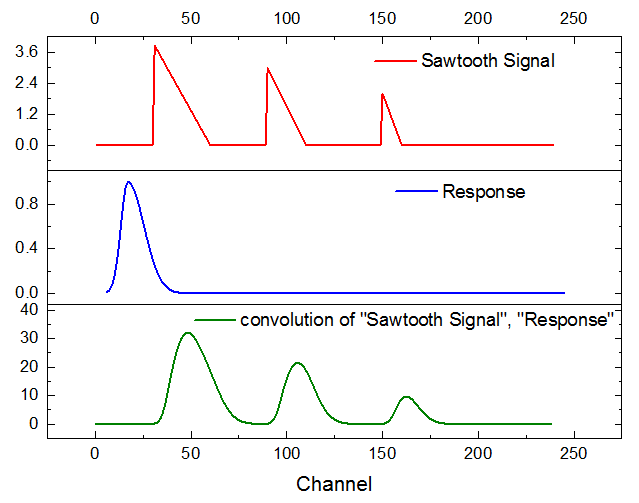With the Convolution tool, you can convolute the signal with response in a Linear or Circular way. You can also elect to normalize or wrap response.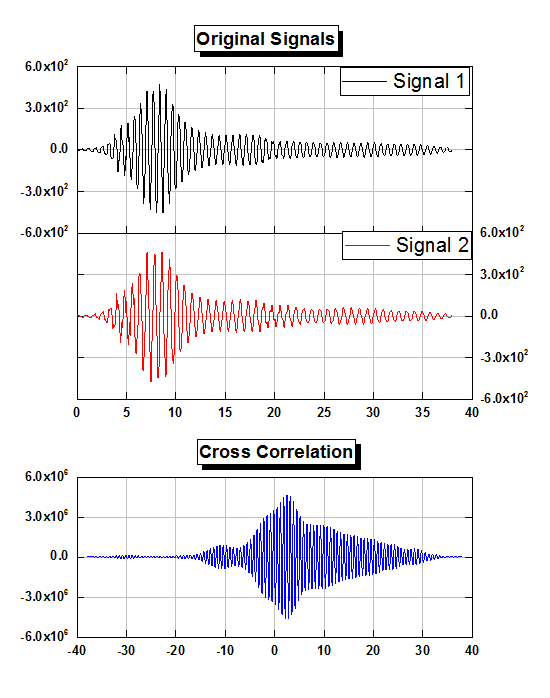The linear correlation result of Signal 1 and 2. The correlation tool in Origin provides both linear and circular correlation types, and an option whether to normalize the correlation coefficient.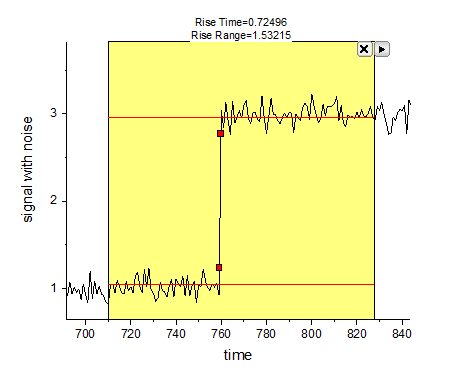With the Rise Time Gadget, you simply drag to position and resize the ROI object to cover the target signal range and the resulting rise time, rise range, etc., will display in real time.

## Apps

Extend Signal Processing functionality of Origin by installing free Apps from our File Exchange site. A selection of Signal Processing Apps are displayed below.